Refer to our Texas Go Math Grade 4 Answer Key Pdf to score good marks in the exams. Test yourself by practicing the problems from Texas Go Math Grade 4 Module 5 Assessment Answer Key.

Concepts and skills

Use the model to write an equation.

Question 1.latex]\frac{3}{5}-\frac{2}{5}[/latex] = $$\frac{1}{5}$$
Explanation:
By using the fraction strip completed the equation

Question 2.latex]\frac{5}{6}-\frac{1}{6}[/latex] = $$\frac{4}{6}$$
Explanation:
By using fraction strip completed the equation

Use the model to find the sum.

Question 3.
$$\frac{3}{8}+\frac{2}{8}$$ = ___________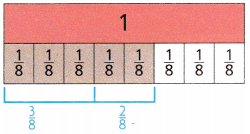$$\frac{3}{8}+\frac{2}{8}$$ = $$\frac{5}{8}$$
Explanation:
By using the fraction strip found the sum of fractions

Question 4.
$$\frac{4}{10}+\frac{5}{10}$$ = ___________$$\frac{4}{10}+\frac{5}{10}$$ = $$\frac{9}{10}$$
Explanation:
By using the fraction strip found the sum of two fractions.

Use the model to find the difference.

Question 5.
$$\frac{5}{6}-\frac{3}{6}$$ = $$\frac{}{6}$$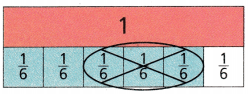$$\frac{5}{6}-\frac{3}{6}$$ = $$\frac{2}{6}$$
Explanation:
By using the fraction strip found the difference of two fractions.

Question 6.
$$\frac{7}{10}-\frac{4}{10}$$ = $$\frac{}{10}$$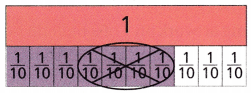$$\frac{7}{10}-\frac{4}{10}$$ = $$\frac{3}{10}$$
Explanation:
By using the fraction strip found the difference of two fractions

Find the sum or difference. Use fraction strips or a number line.

Question 7.
$$\frac{9}{12}-\frac{7}{12}$$ = ____________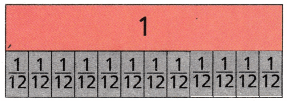$$\frac{9}{12}-\frac{7}{12}$$ = $$\frac{2}{12}$$
Explanation:
By using the fraction strip found the difference of two fractions

Question 8.
$$\frac{2}{3}+\frac{1}{3}$$ = ____________
$$\frac{2}{3}+\frac{1}{3}$$ = $$\frac{3}{3}$$ = 1
Explanation:
By using the fraction strip found the sum of the fractions

Question 9.
$$\frac{1}{5}+\frac{3}{5}$$ = ____________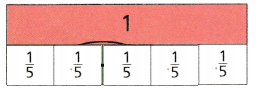$$\frac{1}{5}+\frac{3}{5}$$ = $$\frac{4}{5}$$
Explanation:
By using the fraction strip found the sum of the fractions

Question 10.
$$\frac{2}{6}+\frac{2}{6}$$ = ____________$$\frac{2}{6}+\frac{2}{6}$$ = $$\frac{4}{6}$$
Explanation:
By using the fraction strip found the sum of two fractions

Question 11.
$$\frac{4}{4}-\frac{2}{4}$$ = ____________
$$\frac{4}{4}-\frac{2}{4}$$ =$$\frac{2}{4}$$
Explanation:
By using the fraction strip found the sum of two fractions

Question 12.
$$\frac{7}{8}-\frac{4}{8}$$ = ____________
$$\frac{7}{8}-\frac{4}{8}$$ = $$\frac{3}{8}$$
Explanation:
By using the fraction strip found the difference of two fractions

Question 13.
Tyrone mixed $$\frac{7}{12}$$ quart of red paint with $$\frac{1}{12}$$ quart of yellow paint. how much paint does Tyrone have in the mixture?(A) $$\frac{8}{24}$$ quart
(B) $$\frac{6}{12}$$ quart
(C) $$\frac{8}{12}$$ quart
(D) $$\frac{12}{12}$$ quart
Explanation:
$$\frac{7}{12}$$ + $$\frac{1}{12}$$ = $$\frac{8}{12}$$ quart paint that Tyrone have in the mixture.

Question 14.
Jorge lives $$\frac{6}{8}$$ mile from school and $$\frac{2}{8}$$ mile from a ballpark. How much farther does Jorge live from school than from the ballpark?(A) $$\frac{4}{16}$$ miles
(B) $$\frac{4}{8}$$ miles
(C) $$\frac{8}{8}$$ miles
(D) 8 miles
Explanation:
$$\frac{6}{8}$$ – $$\frac{2}{8}$$ = $$\frac{4}{8}$$ miles farther that Jorge live from school than from the ballpark

Question 15.
Eloise hung artwork on $$\frac{2}{5}$$ of a bulletin board. She hung math papers on $$\frac{1}{5}$$ of the same bulletin board. What part of the bulletin hoard does not have artwork or math papers? Use models to help.
(A) $$\frac{1}{10}$$
(B) $$\frac{3}{5}$$
(C) $$\frac{3}{10}$$
(D) $$\frac{2}{5}$$
$$\frac{2}{5}$$ + $$\frac{1}{5}$$ = $$\frac{3}{5}$$
1 – $$\frac{3}{5}$$
= $$\frac{2}{5}$$ part of the bulletin board  not have artwork or math papers